Browse by Topic
Related Topics

# The Circles of Descartes

Somewhere, you’ve likely been forced to learn how fractions work, and how to calculate 2/7 + 2/5. To some extent, fractions have been falling out of favor in the world, losing out to decimals. The New York Stock Exchange gave up fractions on April 9, 2001.

Much of the time, a decimal is okay. Sometimes, though, especially in mathematics, exact values are desired. Instead of a value being 3.00000000…00727…, it is exactly 3. Or exactly 10/35 + 14/35 = 24/35. For fractions themselves, the Farey sequence is quite interesting—the reduced fractions between 0 and 1 where the denominator is less than or equal to a particular value, like 7. For example, the F7 Farey sequence is the the first row in the following block. The next row has the denominator. The third row is twice the reciprocal of the denominator squared. The fourth row is the denominator from the third row.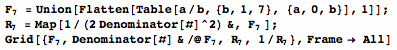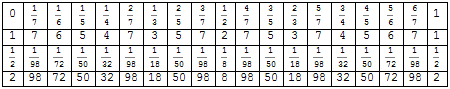In 1938, Lester Ford tried putting circles with radii equal to that block’s third row above their position on the number line. These are now called Ford circles. All these radii have a one on top. Sometimes, it’s nice to use fractions, but it’s better to use integers when possible, so the bend (or curvature) of a circle is 1/radius, as seen in the fourth row. The circle in the middle of the sequence above with radius 1/8 has a bend of 8. Here is a picture of the Ford circles along with their bends, along with Mathematica code for making it. Notice how the circles all exactly touch some other circles. Each circle is tangent to the neighboring circles. Touching = tangent = kissing, when it comes to circles.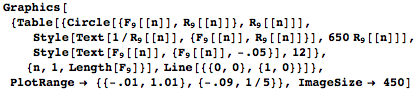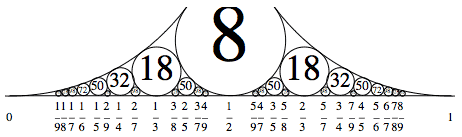Suppose you wanted to find the bend of the circle that would fit between 1/6 and 1/5. One method is to square the sum the square roots of the circle bends above it. Here are some examples.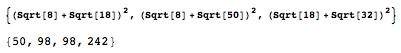Another method is to use the Descartes Circle Theorem. When four circles kiss, or when all four circles touch each other, the bends/curvatures of the circles have an amazing property.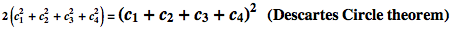That’s for four circles. A line can be thought of as a circle with a bend of 0, or an infinite radius. When given two circles and a line, the elegant formula below finds the possible third circles. This formula can be tested on the circles above 1/3 and 2/5, and the bends of the two circles they both touch are returned.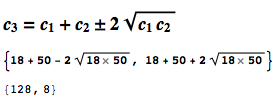Circles don’t have to be in a line, though. Imagine placing circles on the complex plane, the realm of the Mandelbrot set, where (z=x+i y). For the circle with with curvature c1, let z1 be the center point. On the complex plane, when four circles kiss, Mathematica can make use of this expanded Descartes Circle Theorem: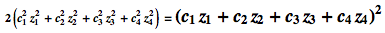Here is that theorem in action. All of the given circles are inside the outer circle, which has a negative bend because the others are inside it. If the first few circles have an integer bend, then all the circles, off to infinity, have integers for 1/radius. Here, the circles are colored mod 12, much like the hours on a clock face. In each grouping, only four colors are possible.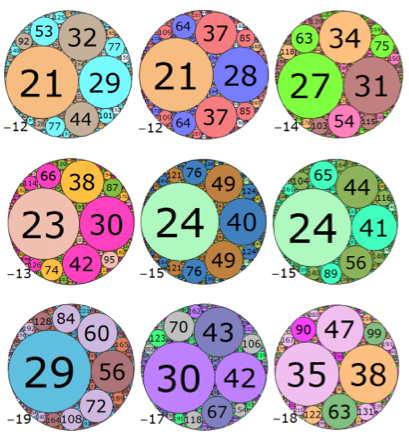And here is a slight modification of The Circles of Descartes Demonstration, with colors added.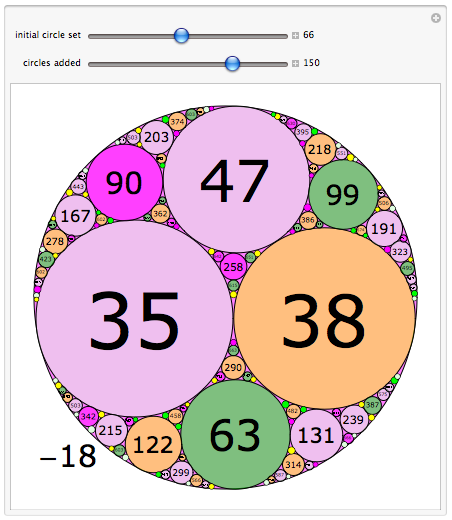With the values given, it’s easy to test the Descartes Circle Theorem. This isn’t a proof, but it’s always a good idea to test something before attempting to prove it: sometimes you’ll find a counterexample right away, and other times you’ll gain insight.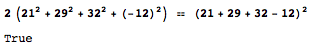I’ll close by mentioning Frederick Soddy, who won a Nobel prize for his study of isotopes but is better known for his research on circles and a poem he published in Nature in 1936. Here’s the first verse:

“The Kiss Precise”
by Frederick Soddy

For pairs of lips to kiss maybe
Involves no trigonometry.
‘Tis not so when four circles kiss
Each one the other three.
To bring this off the four must be
As three in one or one in three.
If one in three, beyond a doubt
Each gets three kisses from without.
If three in one, then is that one
Thrice kissed internally.